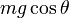# Difference between revisions of "Normal force"

View a complete list of force types

## Definition

### Intensional definition

Normal force, sometimes called normal reaction, is the component of contact force between two bodies acting in a direction perpendicular to the plane of contact (or the tangent plane at the point of contact). It is in the outward direction on both bodies. The magnitudes of the normal force on both bodies are equal and the directions are opposite, in keeping with Newton's third law.

### Tendency-based definition

Normal force is the force exerted by two bodies on each other at a surface of contact that is intended to precisely counteract any tendency the bodies have of moving into each other.

## Situational examples

Example Direction of force on each body Magnitude of force Reason
A block placed on a fixed horizontal surface Upward on the block, downward on the surface Equal to the weight of the block, which is the product of the mass and acceleration due to gravity The net vertical force on the block should be zero
A block placed on a fixed inclined plane with angle$\theta$ with the horizontal Upward/outward on the block, downward/inward on the surface The product$mg \cos \theta$, where$m$ is the mass of the block,$g$ is the acceleration due to gravity.

## Related forces

• Friction: It is the component of contact force along the plane of contact.
• Tension: Tension is a force exerted by a taut string on an object at its end, pulling the object inward.
• Spring force: Spring force is a force exerted by a spring on an object attached at its end. When the spring is extended, the force is inward, analogous to tension, while when the spring is compressed, this acts outward, analogous to normal force.